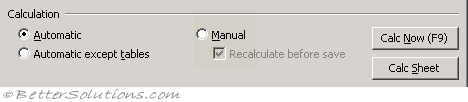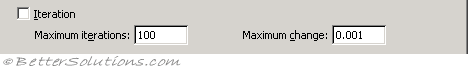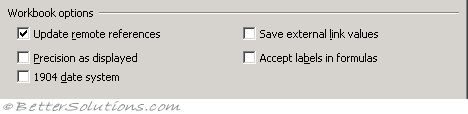# Calculation Tab

Replaced in 2007

### CalculationAutomatic - Calculates all dependent formulas every time you make a change to a value, formula or name. Any workbook that contains formulas will be automatically calculated when it is opened. This is the default calculation setting. (Formulas tab)
Automatic except tables - Calculates all dependent formulas except those in data tables. You can calculate the formulas in data tables by pressing the "Calc Now" button. (Formulas tab - Automatic except for data tables)
Manual - Calculates only when requested by the user. (Formulas tab)
Recalculate before save - Calculates all dependent formulas in the worksheet before it is saved. (Formulas tab - recalculate workbook before saving)
Calculate Now - Calculates all open worksheets, including data tables, and updates all open chart sheets. (Ribbon, Formulas tab)
Calc Sheet - Calculates the active worksheet and any charts and chart sheets linked to this worksheet. (Ribbon, Formulas tab)

### IterationIteration - This can be used to restrict the iteration when using circular references. (Formulas tab - enable iterative calculation)
Maximum iterations - This is the maximum number of iterations used before iteration will stop. The default is 100. (Formulas tab)
Maximum change - The largest number that the change in value, must be less than, before iteration will stop. The smaller the number the more accurate the result. The default is 0.001. (Formulas tab)

### Workbook Options

The following options are all workbook specific and affect all the worksheets in the active workbook.Update remote references - Calculates and updates formulas that include references to other applications and any external functions using a DDE. (Advanced tab, When calculating this workbook - update links to other documents)
Precision as displayed - Changes all the underlying values in your worksheet to be the same as their displayed valued. This will change values permanently from having 15 significant figures to whatever format is displayed. This cannot be undone. (Advanced tab, When calculating this workbook)
1904 date system - Changes the starting date from which all dates are calculated from January 1, 1900, to January 2, 1904. (Advanced tab, When calculating this workbook)
Save external link values - Saves copies of values contained in an external document linked to a Microsoft Excel worksheet. If a worksheet with links to large ranges on an external document requires an unusually large amount of disk space or takes a very long time to open, clearing the Save external link values check box can reduce the disk space and time needed to open the worksheet. (Advanced tab, When calculating this workbook)
Accept labels in formulas - (Removed in 2007). Uses label names in formulas if ranges on your worksheet have row or column labels. Turning this off though mean that any labels that have been used will be replaced with their corresponding cell references. For more information please refer to the Natural Language Formulas page.